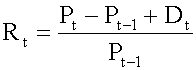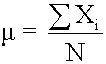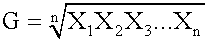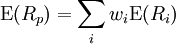### Why should I choose AnalystNotes?

AnalystNotes specializes in helping candidates pass. Period.

##### Subject 1. Major Return Measures
There are various types of return measures.

Holding Period ReturnRefer to Reading 2 for a detailed discussion of this return measure.

Arithmetic or Mean ReturnRefer to Reading 2 for a detailed discussion of this return measure.

Geometric Mean ReturnRefer to Reading 2 for a detailed discussion of this return measure.

Money-Weighted Return or Internal Rate of Return

The dollar-weighted rate of return is essentially the internal rate of return (IRR) on a portfolio. This approach considers the timing and amount of cash flows. It is affected by the timing of cash flows. If funds are added to a portfolio when the portfolio is performing well (poorly), the dollar-weighted rate of return will be inflated (depressed).

The time-weighted rate of return measures the compound growth rate of \$1 initial investment over the measurement period. Time-weighted means that returns are averaged over time. This approach is not affected by the timing of cash flows; therefore, it is the preferred method of performance measurement.

Example

Jayson bought a share of IBM stock for \$100 on December 31, 2000. On December 31, 2001, he bought another share for \$150. On December 31, 2002, he sold both shares for \$140 each. The stock paid a dividend of \$10 per share at the end of each year.

To calculate the dollar-weighted rate of return, you need to determine the timing and amount of cash flows for each year, and then set the present value of net cash flows to be 0: - 100 - 140/(1 + r) + 300/(1 + r)2 = 0. You can use the IRR function on a financial calculator to solve for r to get the dollar-weighted rate of return: r = 17%.

To calculate the time-weighted rate of return:

- Split the overall measurement period into equal sub-periods on the dates of cash flows.

For the first year:
-- beginning price: \$100
-- dividends: \$10
-- ending price: \$150

- For the second year:
-- beginning price: \$300 (150 x 2)
-- dividends: \$20 (10 x 2)
-- ending price: \$280 (140 x 2)

Calculate the holding period return (HPR) on the portfolio for each sub-period: HPR = (Dividends + Ending Price)/Beginning Price - 1. For the first year, HPR1: (150 + 10)/100 - 1 = 0.60. For the second year, HPR2: (280 + 20)/300 - 1 = 0.

Calculate the time-weighted rate of return:
- If the measurement period < 1 year, compound holding period returns to get an annualized rate of return for the year.
- If the measurement period > 1 year, take the geometric mean of the annual returns.Annualized Return

Annualizing returns allows for comparison among different assets and over different time periods.

rannual = (1 + rperiod)c - 1

where c is the number of periods in a year and rperiod is the rate of return per period.

Example

Monthly return: 0.6%. The annualized return is (1 + 0.6%)12 - 1 = 7.44%.

Portfolio Return

The expected return on a portfolio of assets is the market-weighted average of the expected returns on the individual assets in the portfolio.where Rp is the return on the portfolio, Ri is the return on asset i and wi is the weighting of component asset i (that is, the share of asset i in the portfolio).

Other Major Return Measures

1. A gross return is the return before any fees, expense, taxes, etc. A net return is the return after deducting all fees and expenses from the gross return.

2. Different types of investments generate different types of income and have different tax implications. For example, in the U.S. the interest income is fully taxable at an investor's marginal tax rate while capital gains are taxed at a much lower rate. Therefore, many investors therefore use the after-tax return to evaluate mutual fund performance.

3. The nominal return and the real return are two ways to measure how well an investment is performing. The real return takes into consideration the effects of inflation when calculating how much buying power has changed.

4. An investor can also use leverage to amplify his expected return (and risk).

User Comment
synner how do you use IRR function on a TI BAII plus?
jimmymh IRR is a function of CF, input your CF then Push IRR then compute.
rche Can anyone tell me the exact key stroke to solve for the 17% in the IBM example using the BA II Plus?
Evgenia1 CF
C01=-100
F01=1
C02=-140
F02=1
C03=300
IRR CPT
Result: 16.82
yly13 shouldn't it be -100-150/(1+r)+300/(1+r)2
HBomb yly13

There is a \$10 Dividend after the first year also which you add to the \$-150. This gives you \$-140 instead.
KenSemer How do you compute the Time Weighted Rate of Return using BA II Plus?
clyde Hi,
Can anyone tell me for Time-Weighted, in 2nd yr, the beginning price is 300(150x2)? Yr1 has ending \$150. Where does the other 150 come from. Many thanks
akanimo clyde, jayson bought a second IBM share at the end of the year which combines with the initial purchase to give 2 shares, both the new share and the old share will be valued at this same new price i.e. 150 x 2
sdivietr how do I calculate the Time wrr if period < 1 year? Compounding of individual HPR means?????
Taimoor If the return Time Weighted Rate of Return is less than 1 year the compounded annual return is calculated as follows:

e.g a weekly return of 0.2%

(1.002)^52 - 1 = 0.1095 or 10.95%

note: 52 is used as a power because the return is weekly and their are 52 weeks in a year.
achu Geometric Mean formula used with TIME wtd returns, the preferred measure of performance!
HaTran What is different betweent money - weighted and dollar - weighted rates of return???
vinnybozz Dollar Weighted Solution is:

t=0 first purchase: -100
t=1 dividend on 1st share: +10
2nd purchase: -150
t=2 dividend on 2 shares: +20 (10+10)
sale: +280 (140*2)

So at t=0, Solve r:
-100 + (+10-150)/(1 + r) + (+20+280)/(1 + r)2=0
<=> - 100 - 140/(1 + r) + 300/(1 + r)2=0
Gooner7 is there a way to do time-weighted calculation on TI BA II+ ?
DonAnd There is a similar example for calculating Money-Weighted(Dollar-Weghted)rate of return on p.246 in the text.
LONG For dollar-weighted rate of return I can TI BAII as following and get the same result 16.8154%:
CFo=-100
C01=-140
C02=300
IRR=16.8154

and same as:
CFo=0
C01=-100
C02=-140
C03=300
IRR = 16.8154
poomie83 Long, could you explain why 140 is negative? Also why is the cash flow at end of year 2 \$300 when that is the value at end of year 1? And don't we account for the dividends?
TiredHand \$140 is negative because the dude paid \$150 for a second IBM share (unwise) which is -\$150, but he received a \$10 dividend.
\$-150 + \$10 = \$-140.

Not that I could do that question in an exam.
niyongana in which situation liquidity and maturity cannot be investment constraint illustration by using numerical example
ran123 Can someone please show how the dollar weighted return example was calculated manually(step by step)?

Thanks
jonan203 can you rephrase your question?
tshepi van you share how to do it on the HP12c
Callie2 HP12C:
100[CHS][g][CF1] (price of 1st share)
140[CHS][g][CF1] (price of 2nd share - \$10 div)
300[g][CF1] (proceeds plus two \$10 div)
[f][IRR]
=16.8154
bschmitt For BAII Plus, what is F01 and F02 in the calculation please?
schweitzdm F01 and F02 refer to the frequency of cash flows.

If it only happens once, leave it at 1.
Inspector HPR = (Ending price - beginning price + dividends) / beginning price so why do the notes say HPR = (Ending price + dividends)/ beginning price... wtf

HPR = ((MV1 - MV0 + D1 - CF1)/MV0)

Where: MV0 = beginning market value, MV1 = ending market value,
D1 = dividend/interest inflows, CF1 = cash flow received at period end (deposits subtracted, withdrawals added back)
littlecow @Inspector: you missed the -1 part. The notes says HPR = (Dividends + Ending Price)/Beginning Price - 1, this is exactly the same as what you have: HPR = (Ending price - beginning price + dividends) / beginning price
ajshittu HP 12c Platinum Financial Calculator - IRR Calculation
100(CHS)(g)(CF0)
140(CHS)(g)(CFj)
300(g)(CFj)
2(RCL)(n)
(f)(IRR)
kaichan91 Can someone elaborate on the part that talks about inflation/depression of the dollar-weighted rate of return? I'm a little confused about the mathematical relationship of the two.
Rudger I dont understand how the square root of 1.06 is 1.26. I get 1.29. ANy help anyone?
khalifa92 in long time horizons situations?
gjohnub Here's how I do it.
1.He buys \$100 of share on Dec 31, 2000 : so beginning price = \$100
2.He buys \$150 of share on Dec 31, 2001: so ending value = \$150
3. So HPR = (ending- beginning + dividend)/ beginning = (150 - 100 + 10) / 100 = 0.6
4. Then, he sold shares for \$140 on Dec 31, 2002. so ending value = \$140 and beginning value = \$150 from previous year.
5. So HPR = (ending - beginning + dividend) / beginning = (140 - 150 + 10) /150 = 0
6. TWRR = [(1+0.6)(1+0)]^1/2 - 1 ( 1/2 because 2 years ) = 0.26
931129 Go over the calculations here.